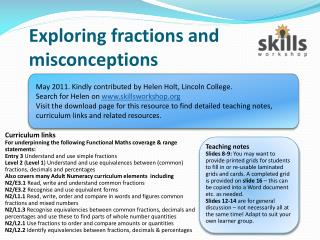DownloadDownload PresentationExploring fractions and misconceptions

# Exploring fractions and misconceptions

Télécharger la présentation## Exploring fractions and misconceptions

- - - - - - - - - - - - - - - - - - - - - - - - - - - E N D - - - - - - - - - - - - - - - - - - - - - - - - - - -
##### Presentation Transcript

1. Exploring fractions and misconceptions May 2011. Kindly contributed by Helen Holt, Lincoln College. Search for Helen on www.skillsworkshop.org Visit the download page for this resource to find detailed teaching notes, curriculum links and related resources. Curriculum links For underpinning the following Functional Maths coverage & range statements: Entry 3 Understand and use simple fractions Level 2 (Level 1) Understand and use equivalences between (common) fractions, decimals and percentages Also covers many Adult Numeracy curriculum elements including N2/E3.1 Read, write and understand common fractions N2/E3.2 Recognise and use equivalent forms N2/L1.1 Read, write, order and compare in words and figures common fractions and mixed numbers N2/L1.3 Recognise equivalencies between common fractions, decimals and percentages and use these to find parts of whole number quantities N2/L2.1 Use fractions to order and compare amounts or quantities N2/L2.2 Identify equivalencies between fractions, decimals & percentages Teaching notes Slides 8-9: You may want to provide printed grids for students to fill in or re-usable laminated grids and cards. A completed grid is provided on slide 16 – this can be copied into a Word document etc. as needed. Slides 12-14 are for general discussion – not necessarily all at the same time! Adapt to suit your own learner group.

2. Week 4 Functional MathsExploring Fractions and Misconceptions. Helen Holt

3. Session Outcomes: • Be able to read, write, order and compare common fractions. • To identify equivalences between fractions, decimals and percent. • To identify the relationship between fractions, decimals and percent. • To explore misconceptions of fractions. Helen Holt

4. What are fractions? • Fractions, decimals and percent are different ways of representing an amount. • I.e. We could say 50% of something, or ½ or 0.5. These are all the samequantity. • Fractions are about sharing. You may like to think of them as ‘parts of a whole’. Helen Holt

5. A fraction describes part of a whole when the whole is cut into equal parts. This pizza has been cut into three equal parts. We call these thirds. A third is written as: Think about two slices. Two slices is two thirds: What are fractions? Helen Holt

6. Writing fractions? • Look at the pictures below. How many parts are these shapes divided into? Represent these shapes as fractions. Helen Holt

7. Converting between fractions, decimals and %: A same value can be written in different forms. For example: ½ 0.5 50% Is the same as: Is the same as: To convert a fraction into a decimal, divide the top of the fraction by the bottom of the fraction: e.g. ½ = 1÷2 = 0.5 To convert a decimal into a percentage, multiply by 100: e.g. 0.5 x 100 = 50% Helen Holt

8. Converting between fractions, decimals and %: Place the fraction, decimal and % cards into the correct blank spaces on the grid. Helen Holt

9. Helen Holt

10. When might we use fractions: When telling the time (e.g. ¼ past). In shop sales (e.g. a 1/3 off, ½ price). When measuring (e.g. ½ a metre). When dividing (e.g. 1 pizza divided between 6 people). In recipes (e.g. half a dozen). When might we use decimals: When working with money. To show probability, or the likelihood of something happening. To show how many whole and part numbers there are to a value (e.g. 3.25 means 3 whole numbers and one quarter of a whole number). When might we use %: Shops use percentages in sales. Banks use them for loan rates, mortgages, savings accounts.. Weather forecasts use them to tell us the chances of rain. To calculate VAT and income tax. Why use fractions, decimals and %? When working in business we may choose between fractions, decimals and %s to make a sale item look more appealing to customers. For example, 20% off may sound more appealing than 1/5 off a sale item, even though they are really the same amount! Helen Holt

11. Sharing Amounts Helen Holt

12. Misconceptions of Fractions: • Can a fraction be bigger than one whole? • Is it possible to have three halves of one object? • Are fractions anything to do with division? • A fraction is a small piece of a whole. Helen Holt

13. Misconceptions of Fractions: • You cannot have a fraction that is bigger than one. • Five is less than six so 1/5 must be smaller than 1/6. • Decimals and fractions are completely different types of numbers. Helen Holt

14. Misconceptions of Fractions: • Every fraction can be written as a decimal. • Every percentage can be written as a decimal and a fraction? • If you add the same number to the top and bottom number of a fraction, the fraction gets bigger in value. Helen Holt

15. Where can we find fractions? Take a look at this article. Notice how fractions are used to explain and analyse the results of the survey. Helen Holt

16. Helen Holt